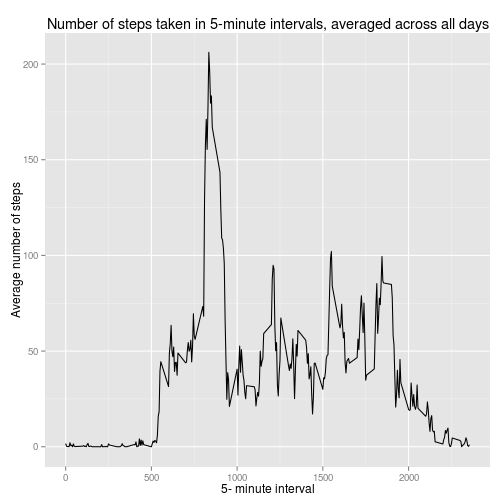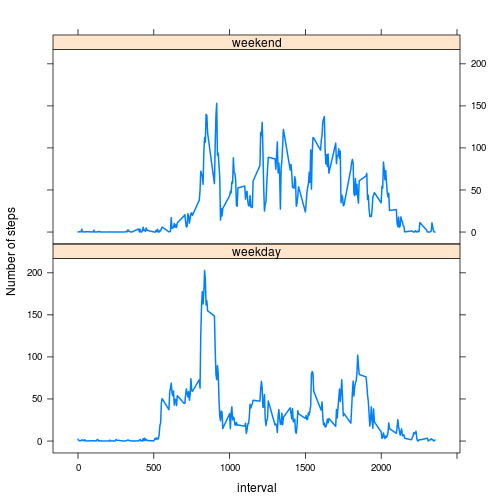# Human activity patterns in “wearables” dataHere is a short analysis of the patterns of human activity taken from a personal activity monitor, or “wearable,” worn by an anonymous individual over the span of two months. From October through November 2012, the monitor recorded data in 5-minute intervals. Full disclosure, this was a neat little assignment from the Coursera class called “Reproducible Research.” I’m assuming it’s ok to post, since this is already on my github (including the data if you are interested) here, but if you’re working on this assignment some time in a future course offering, please don’t use this as your own homework.

First things first, let’s load the activity data into R as a data frame and look at it.

Cool, so we have three variables to work with. Let’s convert the date variable from a factor to a date type.

I’ll want to look at the total steps taken per day, so let’s make a new data frame for total daily steps to make it easier.

## What is the average number of steps taken per day?

Let’s make a simple histogram of the total number of steps taken each day.Now we’ll calculate the mean and median total steps per day.

The mean number of daily steps is 10766, and the median number of daily steps is 10765.

## What does the average daily activity pattern look like?

Let’s manipulate the activity data frame a little so that it is easier to work with.

Now we’ll make a time series plot of the 5-minute interval (x-axis) and the average number of steps taken, averaged across all days (y-axis).Looks like this person does a lot of running around in the morning getting ready before work.

Which 5-minute interval contains the maximum number of steps?

The interval number 835 contains the maximum number of steps. That looks consistent with our plot.

## Imputing missing values

Until now, we just ignored missing values in the data. Let’s try to fill them in with a reasonable guess. First, find the total number of missing values in the dataset.

The steps variable is the only variable with missing values. There are 2304 missing values in the dataset.

Let’s devise a strategy to replace missing values. I will replace the missing values with the average for that interval, since the pattern varies significantly over the course of a day. First, let’s merge the daily pattern data frame with the activity data frame.

Now create a new dataset equal to the original but with the missing data filled in.

Let’s look at a histogram of the total number of steps taken each day and calculate the mean and median total number of steps taken per day with this new imputed data to compare with the original. First I will make a new data frame again for the total daily steps.

Now make the histogram.Now the mean and median total steps are 9371 and 10395, respectively. Imputing missing data has changed these estimates, and in this case, lowers both the mean and median, with a more significant effect on the mean.

## Are there differences in activity patterns between weekdays and weekends?

To look at this, first we’ll create a new factor variable in the dataset with two levels “weekday” and “weekend”

Now let’s look at a panel plot containing a time series plot of the 5-minute interval (x-axis) and the average number of steps taken, averaged across all weekday days or weekend days (y-axis).Yep, it does look like there are differences in activity patterns between weekdays and weekends. During weekdays, there is a peak in activity early in the day (maybe when this guy is getting ready for work or walking to work) and then little activity the rest of the day. During weekends, the activity is consistently slightly higher over the day, but without the peak seen on weekdays.

That was fun! What else could we look at with these data?